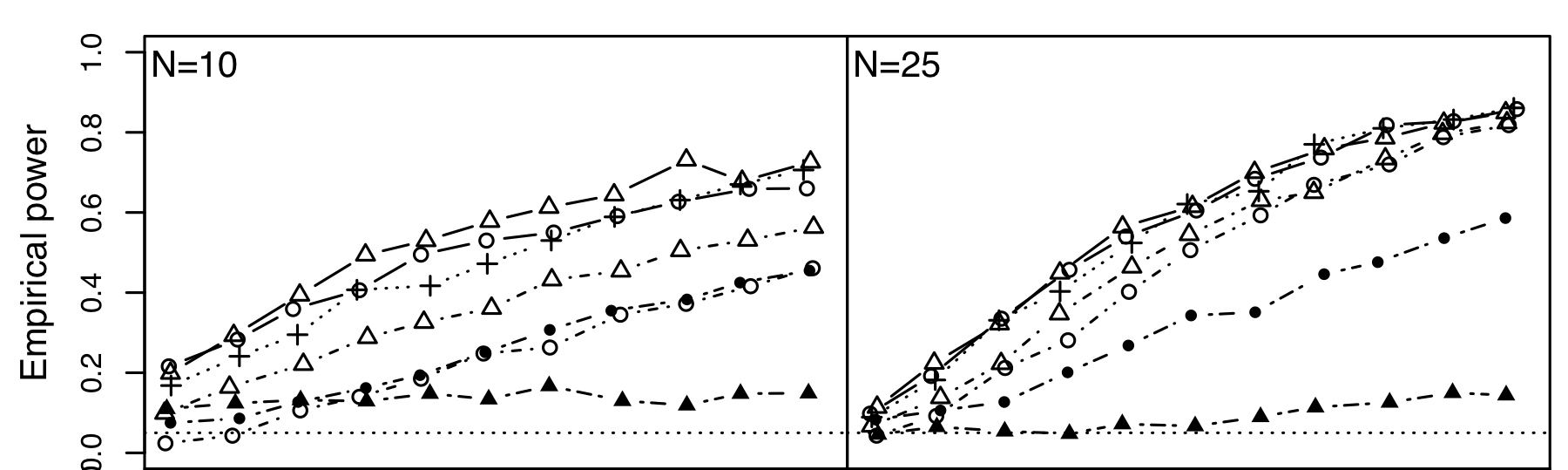Tests for separability in nonparametric covariance operators of random surfaces

Abstract

The assumption of separability of the covariance operator for a random image or hypersurface can be of substantial use in applications, especially in situations where the accurate estimation of the full covariance structure is unfeasible, either for computational reasons, or due to a small sample size. However, inferential tools to verify this assumption are somewhat lacking in high-dimensional or functional data analysis settings, where this assumption is most relevant. We propose here to test separability by focusing on K-dimensional projections of the difference between the covariance operator and a nonparametric separable approximation. The subspace we project onto is one generated by the eigenfunctions of the covariance operator estimated under the separability hypothesis, negating the need to ever estimate the full nonseparable covariance. We show that the rescaled difference of the sample covariance operator with its separable approximation is asymptotically Gaussian. As a by-product of this result, we derive asymptotically pivotal tests under Gaussian assumptions, and propose bootstrap methods for approximating the distribution of the test statistics. We probe the finite sample performance through simulations studies, and present an application to log-spectrogram images from a phonetic linguistics dataset.

Type
Publication
Annals of Statistics, (45)4